# How to plot¶

## Authors:¶

Basic Plotting with Matplotlib (Scatterplot, Barplot, Heatmap, Boxplot, etc.) Cartopy and Map plotting Plotting Contourplot using Precipitation data Choosing colorbars Wind direction (u and v) Making a Skew-T with wind barbs using MetPy Color maps in Matplotlib and creating customized color bar Interactive plotting

Suggestions 23 june

• global plot -- Any data (SST better) with cartopy

# 1. Basic Plotting with Matplotlib (Scatterplot, Barplot, Heatmap, Boxplot, etc.)¶

## What is Matplotlib?¶

Matplotlib is a Python library for creating high-quality visualizations and plots of data.It is the most well-known of all the python visualization packages and provides a variety of capabilities such as:

• Matplotlib can gernerate high-quality graphics in a number of file types including PDF, SVF, JPG, PNG, BMP and GIF.

• Matplotlib include line plots, scatter plots, histograms, bar charts, pie charts, and box plots.

• Additionally, it enables 3D charting, and many additional libraries, such as pandas and Seaborn, have been built on top of it to provide access to Matplotlib's capabilities with less code.

### Some basic functions¶

Let's have a look at some of the basic function that are often used in matplotlib.

Function Description
plt.plot() Creates line plots to represent data.
plt.scatter() Generates scatter plots to display individual data points.
plt.bar() Creates bar plots to visualize categorical data.
plt.hist() Generates histograms to display the distribution of numerical data.
plt.boxplot() Creates boxplots to show the distribution and outliers of a dataset.
plt.pie() Generates pie charts to display proportions or percentages of categorical data.
plt.imshow() Displays images or heatmaps using arrays of data.
plt.show() it displays the created plots
plt.xlabel() This function is used to add a label to the x-axis of the plot.
plt.ylabel() This function is used to add a label to the y-axis of the plot.
plt.title() This function is used to add a title to the plot.
plt.legend() Adds a legend to the plot to identify plotted elements.
plt.xticks() This function is used to set the tick locations and labels for the x-axis.
plt.yticks() This function is used to set the tick locations and labels for the y-axis.
plt.grid() This function is used to add a grid to the plot.
plt.xlim() Sets the limits for the x-axis.
plt.ylim() Sets the limits for the y-axis.
plt.annotate() it is use to write comments on the graph at the specified position
plt.figure(figsize = (x, y)) whenever we want the result to be displayed in a separate window we use this command, and figsize argument decides what will be the initial size of the window that will be displayed after the run
plt.subplot(r, c, i) it is used to create multiple plots in the same figure with r signifies the no of rows in the figure, c signifies no of columns in a figure and i specifies the positioning of the particular plot

These are some common functions used in matplotlib plotting.

### Importing Matplotlib¶

To use matplotlib we need to import it.We will use standard shorthand for matplotlib imports to make things simpler.

In [ ]:
import matplotlib.pyplot as plt


Here we have imported the pyplot module from the matplotlib library as plt.This is a common convention to simplify the usage of pyplot functions throughout the code.

Basically, we'll be using **plt** instead of **matplotlib.pyplot**

### Scatterplot¶

Step by step guide to create a scatterplot:

**Step 1:**

Firstly, we'll import the necessary packages.We'll use matplotlib for plotting and numpy to produce data.

**Step 2:** Then we'll gernerate random data using numpy to plot.Here,we'll use Numpy's random.normal() function to generate 100 random numbers for two variables:x and y

**Step 3:** In this step we'll plot the data using matplotlib's scatter() function to create a scatter plot of the x and y data points.

Syntax of scatterplot :

matplotlib.pyplot.scatter(x, y, s=None, c=None, marker=None, cmap=None, norm=None, vmin=None, vmax=None, alpha=None, linewidths=None, *, edgecolors=None, plotnonfinite=False, data=None, **kwargs)

**Step 4:** Finally, we use the show() function to display the plot.

In [ ]:
# import packages
import matplotlib.pyplot as plt
import numpy as np

# generate random data
x = np.random.normal(size=100)
y = np.random.normal(size=100)

# plot the data
plt.scatter(x, y)

# show the plot
plt.show()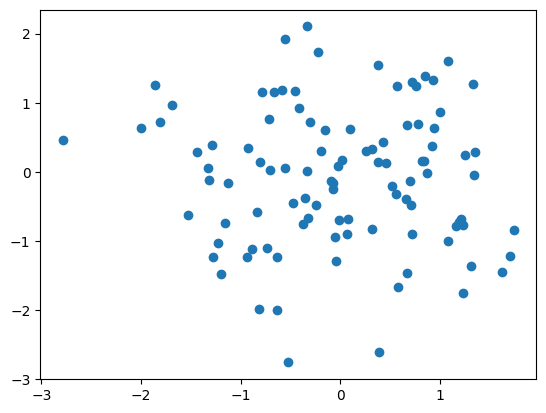### Barplot¶

Step by step guide to create a scatterplot:

**Step 1:** Firstly, we'll import the necessary packages.We'll use matplotlib for plotting and numpy to produce data.

**Step 2:**Then we'll gernerate random data using numpy to plot.Here,we'll use Numpy's NumPy's random.randint() function to generate a list of 8 random integers between 1 and 150.

**Step 3:** In this step we'll use the bar() function from pyplot to create a bar graph. The range(len(data)) is used to generate a sequence of indices corresponding to each data point, and data represents the height of each bar. The color parameter is set to '#900C3F' to specify the color of the bars.

Syntax of bar plot:

matplotlib.pyplot.bar(x, height, width=0.8, bottom=None, *, align='center', data=None, **kwargs)

**Step 4:**Finally, we use the show() function to display the plot.

In [ ]:
#Importing packages
import matplotlib.pyplot as plt
import numpy as np
#Creating random list of 8 numbers.
data = list(np.random.randint(1, 150, 8))
#Plotting the horizontal bar.
plt.bar(range(len(data)), data, color='#900C3F')
#showing the plot
plt.show()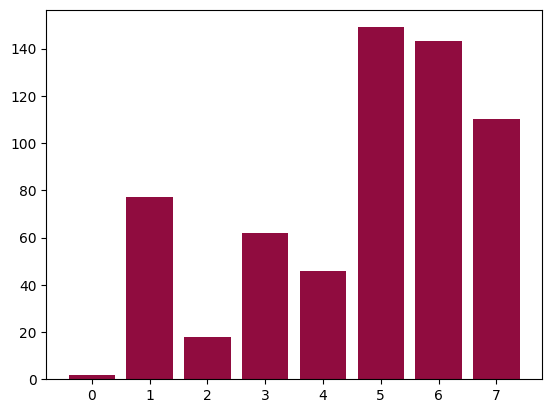### we can also create a horizontal bar chart.¶

In [ ]:
#Importing packages
import matplotlib.pyplot as plt
import numpy as np
#Creating random list of 8 numbers.
data = list(np.random.randint(1, 150, 8))
#Plotting the horizontal bar.
plt.barh(range(len(data)), data, color='#900C3F')
#showing the plot
plt.show()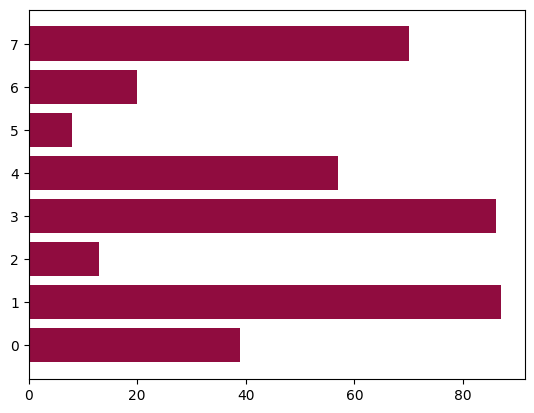### Heatmap¶

A heatmap is a graphical representation of data where values are represented as colors in a two-dimensional grid. Heatmaps are particularly useful for visualizing patterns, correlations, or distributions in data matrices.

Let's see a heatmap**Explanation:**

Here we see a heatmap representing the harvest data of local farmers. Each cell in the heatmap corresponds to a combination of a vegetable and a farmer, with the color intensity representing the harvest amount. The color intensity of each cell gives us the values of harvest with help of color bar included.

#### Let's make a simple Heatmap:¶

Step by step guide to create heatmap:

**Step 1:** Firstly, we'll import the necessary packages.We'll use matplotlib for plotting and numpy to produce data.

**Step 2:**Then we'll gernerate random data using numpy to plot.Here,we'll use Numpy's NumPy's np.random.rand() function to generate a 10x10 random matrix.

**Step 3:** In this step we'll use the imshow() function from pyplot to create a heatmap.The data matrix is used as the input data. The cmap parameter is set to autumn to use the autumn color map.

Syntax of Heat map:

matplotlib.pyplot.imshow(X, cmap=None, alpha=None)
X :- this is input data matrix which is to be displayed
cmap :- Colormap we use t0 dispay the heatmap
alpha :- it specifies the opacity or transpiracy of the heatmap

**Step 4:** a color bar is then added.

**Step 5:**we set the title and labels.

**Step 6:**Finally, we use the show() function to display the plot.

In [ ]:
import matplotlib.pyplot as plt
import numpy as np

# Generate random data
data = np.random.rand(10, 10)  # Generate a 10x10 random matrix

# Create a heatmap
plt.imshow(data, cmap='autumn')

plt.colorbar()

# Set title and labels
plt.title('Heatmap Example')
plt.xlabel('X-axis')
plt.ylabel('Y-axis')

# Show the plot
plt.show()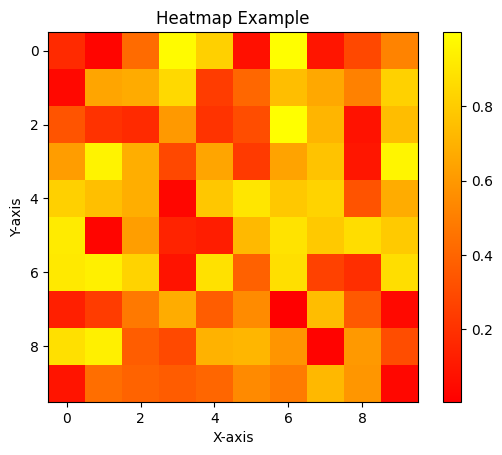### Boxplot¶

A boxplot also known as a box and whisker plot is a graphical representation of the distribution of a dataset.

• It can summarizes key statistical measures such as the median,quartiles, and potential outliers. *The boxplot provides a compact way to visualize the spread and skewness of the data.

It has the following component:**Box:** The box represents the interquartile range (IQR), which contains the middle 50% of the data. The bottom edge of the box represents the 25th percentile (lower quartile), while the top edge represents the 75th percentile (upper quartile). The line inside the box represents the Median.

**Whiskers:** The whiskers extend from the box to the minimum and maximum non-outlier data points. They give an idea of the range of the dataset, excluding any outliers.

**Outliers:** Individual data points that are considered outliers are plotted as individual points outside the whiskers. They are typically defined as points that fall outside a certain multiple of the IQR from the edges of the box.By default, the range is set to 1.5 times the IQR.

Step by step guide to create a boxplot:

**Step 1:** Firstly, we'll import the necessary packages.We'll use matplotlib for plotting and numpy to produce data.

**Step 2:**Then we'll gernerate random dataset using numpy to plot.Here,we'll use Numpy's NumPy's random.normal() function where the loc parameter specifies the mean of the distribution, which is set to 0 in this case. The scale parameter specifies the standard deviation of the distribution, which is set to 1. The size parameter determines the number of data points in the dataset, which is set to 100.Then we added some outliers to data and created the final data.

**Step 3:** In this step we'll use the boxplot() function from pyplot. The data array is passed as the argument, which contains the dataset to be visualized.

Syntax of boxplot:

matplotlib.pyplot.boxplot(x, notch=None, sym=None, vert=None, whis=None, positions=None, widths=None, patch_artist=None, bootstrap=None, usermedians=None, conf_intervals=None, meanline=None, showmeans=None, showcaps=None, showbox=None, showfliers=None, boxprops=None, labels=None, flierprops=None, medianprops=None, meanprops=None, capprops=None, whiskerprops=None, manage_ticks=True, autorange=False, zorder=None, capwidths=None, *, data=None)

**Step 4:**We add title and labels.

**Step 5:**Finally, we use the show() function to display the plot.

In [ ]:
import matplotlib.pyplot as plt
import numpy as np

# Generating random data
data = np.random.normal(loc=0, scale=1, size=100)

outliers = np.array([-3, 3])  # Example outliers
# Final data
data_with_outliers = np.concatenate((data, outliers))

# Create a boxplot
plt.boxplot(data_with_outliers)

# Set title and labels
plt.title('Boxplot Example')
plt.xlabel('Data')

# Show the plot
plt.show()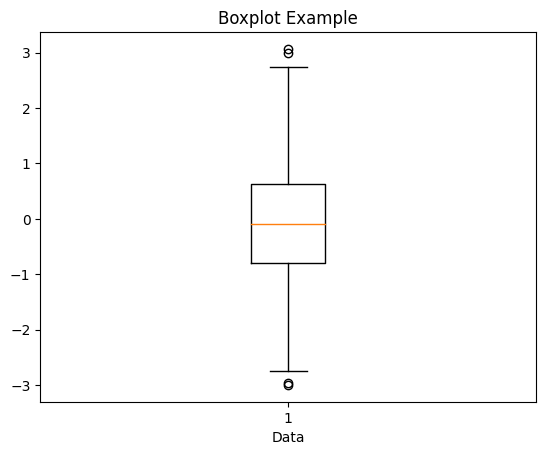In [ ]:



# 2. Cartopy and Map plotting¶

Cartopy is a Python package designed for geospatial data processing in order to make it easier to work with and analyze data that is related to the Earth's surface. It is built on top of several other popular scientific Python packages such as NumPy, Matplotlib, and Shapely, and provides a way to create maps, plot data on maps, and perform various geographical data analysis tasks.

Please note that when using cartopy in colab, it may sometimes crash. To avoid errors, please run the following cell. You can ignore the contents of the cell, and simply copy and paste the code whenever you need to use cartopy. After successfully installing all necessary components, please restart the runtime.

Runtime --> Restart Runtime --> do not run again after restart

In [ ]:
# Run this cell when you are in colab and then restart runtime

!apt-get install libproj-dev proj-data proj-bin
!apt-get install libgeos-dev
!pip install cython
!pip install cartopy

!apt-get -qq install python-cartopy python3-cartopy
!pip uninstall -y shapely    # cartopy and shapely aren't friends (early 2020)
!pip install shapely --no-binary shapely

Reading package lists... Done
Building dependency tree
libproj-dev set to manually installed.
proj-data set to manually installed.
The following NEW packages will be installed:
proj-bin
0 upgraded, 1 newly installed, 0 to remove and 38 not upgraded.
Need to get 170 kB of archives.
After this operation, 485 kB of additional disk space will be used.
Get:1 http://ppa.launchpad.net/ubuntugis/ppa/ubuntu focal/main amd64 proj-bin amd64 7.2.1-1~focal0 [170 kB]
Fetched 170 kB in 0s (425 kB/s)
Selecting previously unselected package proj-bin.
(Reading database ... 122541 files and directories currently installed.)
Preparing to unpack .../proj-bin_7.2.1-1~focal0_amd64.deb ...
Unpacking proj-bin (7.2.1-1~focal0) ...
Setting up proj-bin (7.2.1-1~focal0) ...
Processing triggers for man-db (2.9.1-1) ...
Building dependency tree
libgeos-dev set to manually installed.
0 upgraded, 0 newly installed, 0 to remove and 38 not upgraded.
Looking in indexes: https://pypi.org/simple, https://us-python.pkg.dev/colab-wheels/public/simple/
Requirement already satisfied: cython in /usr/local/lib/python3.10/dist-packages (0.29.34)
Looking in indexes: https://pypi.org/simple, https://us-python.pkg.dev/colab-wheels/public/simple/
Collecting cartopy
━━━━━━━━━━━━━━━━━━━━━━━━━━━━━━━━━━━━━━━━ 10.9/10.9 MB 86.4 MB/s eta 0:00:00
Installing build dependencies ... done
Getting requirements to build wheel ... done
Requirement already satisfied: numpy>=1.18 in /usr/local/lib/python3.10/dist-packages (from cartopy) (1.22.4)
Requirement already satisfied: matplotlib>=3.1 in /usr/local/lib/python3.10/dist-packages (from cartopy) (3.7.1)
Requirement already satisfied: shapely>=1.6.4 in /usr/local/lib/python3.10/dist-packages (from cartopy) (2.0.1)
Collecting pyshp>=2.1 (from cartopy)
━━━━━━━━━━━━━━━━━━━━━━━━━━━━━━━━━━━━━━━━ 46.5/46.5 kB 5.3 MB/s eta 0:00:00
Collecting pyproj>=3.0.0 (from cartopy)
━━━━━━━━━━━━━━━━━━━━━━━━━━━━━━━━━━━━━━━━ 7.7/7.7 MB 55.0 MB/s eta 0:00:00
Requirement already satisfied: contourpy>=1.0.1 in /usr/local/lib/python3.10/dist-packages (from matplotlib>=3.1->cartopy) (1.0.7)
Requirement already satisfied: cycler>=0.10 in /usr/local/lib/python3.10/dist-packages (from matplotlib>=3.1->cartopy) (0.11.0)
Requirement already satisfied: fonttools>=4.22.0 in /usr/local/lib/python3.10/dist-packages (from matplotlib>=3.1->cartopy) (4.39.3)
Requirement already satisfied: kiwisolver>=1.0.1 in /usr/local/lib/python3.10/dist-packages (from matplotlib>=3.1->cartopy) (1.4.4)
Requirement already satisfied: packaging>=20.0 in /usr/local/lib/python3.10/dist-packages (from matplotlib>=3.1->cartopy) (23.1)
Requirement already satisfied: pillow>=6.2.0 in /usr/local/lib/python3.10/dist-packages (from matplotlib>=3.1->cartopy) (8.4.0)
Requirement already satisfied: pyparsing>=2.3.1 in /usr/local/lib/python3.10/dist-packages (from matplotlib>=3.1->cartopy) (3.0.9)
Requirement already satisfied: python-dateutil>=2.7 in /usr/local/lib/python3.10/dist-packages (from matplotlib>=3.1->cartopy) (2.8.2)
Requirement already satisfied: certifi in /usr/local/lib/python3.10/dist-packages (from pyproj>=3.0.0->cartopy) (2022.12.7)
Requirement already satisfied: six>=1.5 in /usr/local/lib/python3.10/dist-packages (from python-dateutil>=2.7->matplotlib>=3.1->cartopy) (1.16.0)
Building wheels for collected packages: cartopy
Building wheel for cartopy (pyproject.toml) ... done
Created wheel for cartopy: filename=Cartopy-0.21.1-cp310-cp310-linux_x86_64.whl size=11102773 sha256=179a617d0278069c1ed854f1f61cebc93e56f87dade36cdce47639a96b73b842
Stored in directory: /root/.cache/pip/wheels/30/b0/1a/1c1909e00c76653dc4e2ff48555257c0eb2d1698280c8d9955
Successfully built cartopy
Installing collected packages: pyshp, pyproj, cartopy
Successfully installed cartopy-0.21.1 pyproj-3.5.0 pyshp-2.3.1
E: Unable to locate package python-cartopy
Found existing installation: shapely 2.0.1
Uninstalling shapely-2.0.1:
Successfully uninstalled shapely-2.0.1
Looking in indexes: https://pypi.org/simple, https://us-python.pkg.dev/colab-wheels/public/simple/
Collecting shapely
━━━━━━━━━━━━━━━━━━━━━━━━━━━━━━━━━━━━━━ 275.5/275.5 kB 17.4 MB/s eta 0:00:00
Installing build dependencies ... done
Getting requirements to build wheel ... done
Installing backend dependencies ... done
Requirement already satisfied: numpy>=1.14 in /usr/local/lib/python3.10/dist-packages (from shapely) (1.22.4)
Building wheels for collected packages: shapely
Building wheel for shapely (pyproject.toml) ... done
Created wheel for shapely: filename=shapely-2.0.1-cp310-cp310-linux_x86_64.whl size=969692 sha256=35bddb8214194f4870c91d517c12419d610404ae1742225bc75791ef2390beda
Stored in directory: /root/.cache/pip/wheels/07/bd/06/4e979fa263bca266484ee65f5aab8e6b1c9b20f8caa6f2d7da
Successfully built shapely
Installing collected packages: shapely
Successfully installed shapely-2.0.1

In [ ]:
# importing libraries

import warnings
warnings. filterwarnings("ignore")

import matplotlib.pyplot as plt

# importing cartopy
import cartopy.feature as cf
import cartopy.crs as ccrs
import cartopy.mpl.ticker as cticker


Let's start by creating a basic map using cartopy. To do this, we first need to define an axes and a projection. Once the projection is defined, we can add coastlines to the map, specifying the linewidth and color. We'll delve deeper into projections later on.

In [ ]:
map = plt.axes(projection=ccrs.PlateCarree()) # defining axes and projection* and assigned it to map

Out[ ]:
<cartopy.mpl.feature_artist.FeatureArtist at 0x7ff02c14e3b0>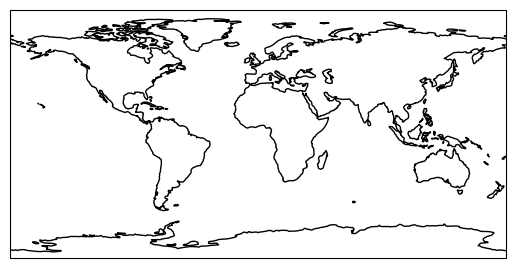#### Add Features to the Map¶

We add features by calling the add_feature() method on-axis object and passing a feature object available from the cartopy.feature module.

We can add the following features (part of cartopy.feature) to our map:

• COASTLINE: Adds coastline around all continents.
• LAND: Adds land on top of world map.
• LAKES: Adds big lakes of the world.
• BORDERS: Adds country borders for the whole world.
• OCEAN: Adds ocean with a color.
• RIVERS: Adds big rivers of the world.
In [ ]:
# Adding more features and add stock images
plt.figure(figsize=(12,12))
map4 = plt.axes(projection=ccrs.PlateCarree())


<matplotlib.image.AxesImage at 0x7ff02c1acaf0>